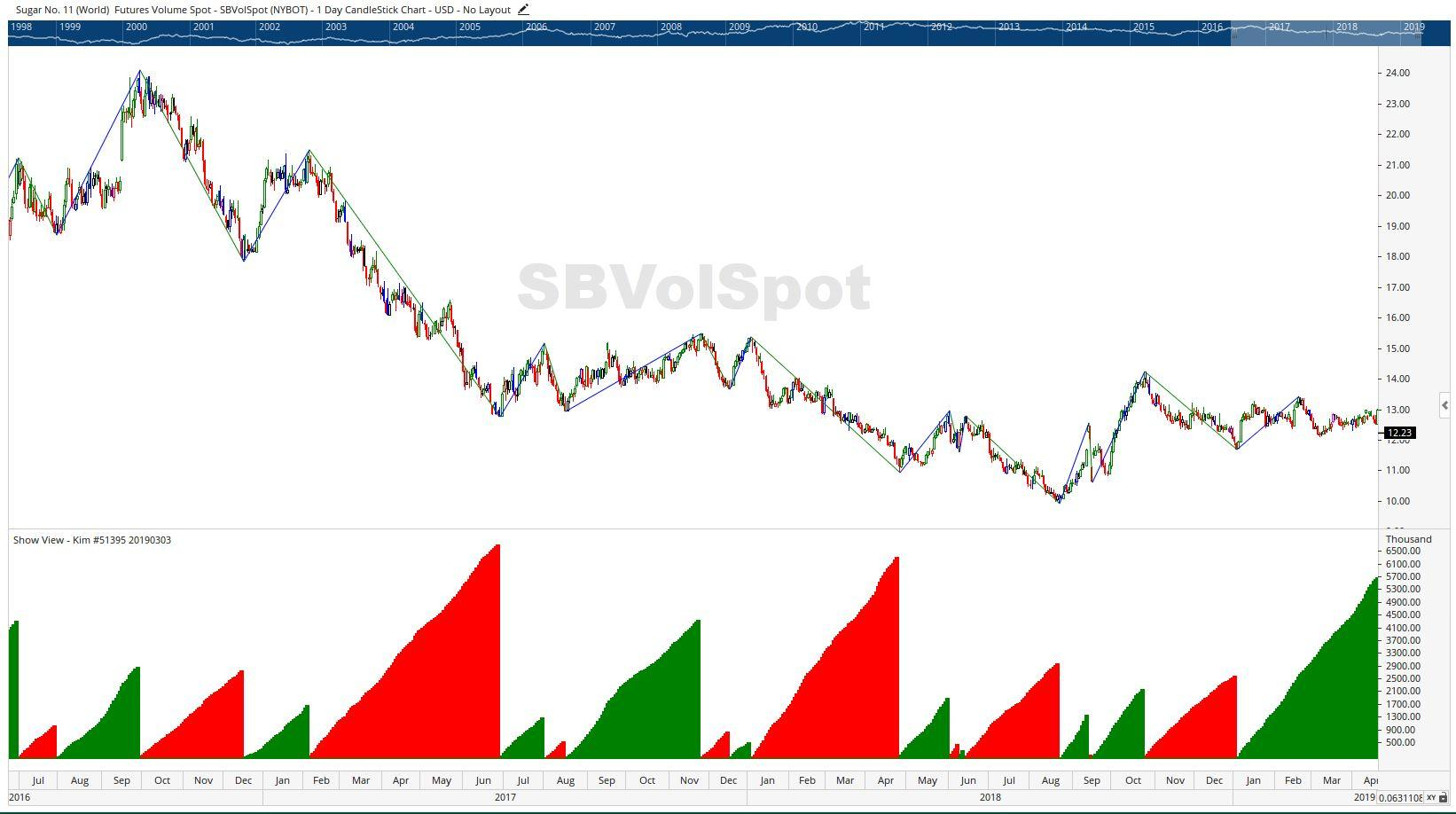Select Page

# Reply To: Variant Of Weis Wave

Optuma Forums Optuma Scripting Variant Of Weis Wave Reply To: Variant Of Weis Wave

#52965

Hi Kim,

Apologies for not getting back to you sooner, but here is a revised version of your script. I’ve commented out the parts of your script and added my changes in bold italics so you can see what I’ve done.

// Kim #51395 20190303

//Volume Between Swings

//PSW = PERCENTSWING(PERCENT=5.0, DEFAULT=SwingEnd, COLOURSTYLE=Up/Down);

PSW = PERCENTSWING(PERCENT=10, DEFAULT=SwingList, COLOURSTYLE=Up/Down);

PSWStart= SWINGSTART(PSW);

//PSWEnd = SWINGEND(PSWEnd);

PSWEnd = SWINGEND(PSW);

Rng = PSWStart – PSWEnd;

// Down Swings bars

//Sig1 = if((TIMESINCESIGNAL(PSW) > 0 and Rng > 0) , 1, 0);

Sig1 = if((TIMESINCESIGNAL(PSWStart) > 0 and Rng > 0) , 1, 0);

//Sig2 = IF(TIMESINCESIGNAL(PSWEnd) < 1 and Rng < 0, 1, 0) ;

Sig2 = IF(TIMESINCESIGNAL(PSWStart) > 0 and Rng < 0, -1, 0) ;

//Sig3= If(Sig1 or Sig2, 1, 0);

// Up Swing Bars

//Sig4 = if((TIMESINCESIGNAL(PSW) > 0 and Rng < 0), 1, 0);

//Sig5= IF(TIMESINCESIGNAL(PSWEnd) < 1 and Rng > 0, 1, 0) ;

//Sig6= If(Sig4 or Sig5, 1, 0);

// Plot the Down trend Volume

//Plot1 = If(Sig3, ACCSINCESIGNAL(Vol(), Sig1), 0) ;

Plot1 = If(Sig1, ACCSINCESIGNAL(Vol(), Sig1), 0) ;

Plot1.Colour = Red;

Plot1.Plotstyle = Histogram;

Plot1.LineWidth = 5;

Plot1.LineStyle = Solid;

// Plot the Up trend Volume

//Plot2 = If(Sig6, ACCSINCESIGNAL(Vol(), Sig4), 0) ;

Plot2 = If(Sig2, ACCSINCESIGNAL(Vol(), Sig2), 0) ;

Plot2.Colour = Green; Plot2.Plotstyle = Histogram;

Plot2.LineWidth = 5;

Plot2.LineStyle = Solid;

Here what the resulting plot looks like (with PercentSwing = 10%):Is this the result you are after?

Cheers

Trevor

The Auld Tyma from